C Program to Print Array Elements at Even Position

In this tutorial you will learn about the C Program to Print Array Elements at Even Position and its application with practical example.

C Program to Print Array Elements at Even Position

In this tutorial, we will learn to create a C program that will Print Array Elements at Even Positions in C programming.

Prerequisites

Before starting with this tutorial we assume that you are best aware of the following C programming topics:

• Operators in C Programming.
• Basic Input and Output function in C Programming.
• Basic C programming.
• For loop in C programming.
• Conditional Statements in C programming.
• Arithmetic Operators in C programming.

Print Array Elements at Even Positions

As we all know array is a collection of similar data type elements. In an array, only one variable is declared which can store multiple values. First will take the number of elements of an array from the user. Then will take the elements from the user for the array. And at last, we will Print Array Elements at Even Positions C Programming Language.

With the help of this program, we can Print Array Elements at Even Positions.

Output:-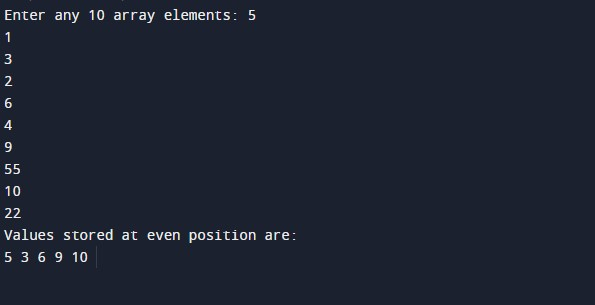In the above program, we have first initialized the required variable.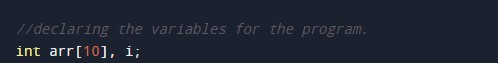• arry = it will hold the string value.
• i = it will hold the integer value for controlling the loop.

Getting the elements in the array for printing the Even index values.For Loop for input.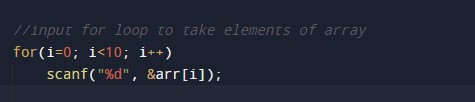Calculating the array elements of the Even index values.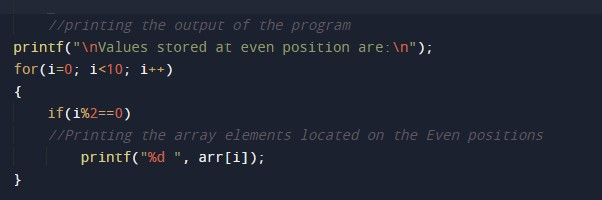Printing the output for the Even Index values.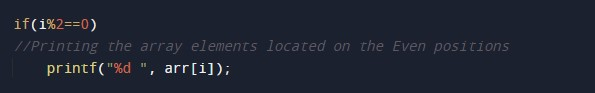In this tutorial we have learn about the C Program to Print Array Elements at Even Position and its application with practical example. I hope you will like this tutorial.